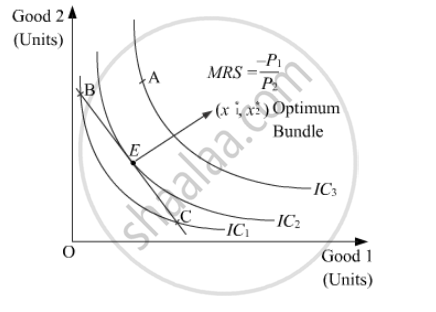Advertisement Remove all ads

# What Are the Conditions of Consumer’S Equilibrium Under the Indifference Curve Approach? What Changes Will Take Place If the Conditions Are Not Fulfilled to Reach Equilibrium? - Economics

Advertisement Remove all ads
Advertisement Remove all ads
Advertisement Remove all ads

What are the conditions of consumer’s equilibrium under the indifference curve approach? What changes will take place if the conditions are not fulfilled to reach equilibrium?

Advertisement Remove all ads

#### Solution

According to the Indifference Curve Approach, a consumer attains equilibrium at the point where the budget line is tangent to the indifference curve and the IC is convex to the origin at the point of tangency. This optimum point is characterised by the following equality:

|(-dy)/dx|=|MRS|=|(-P_1)/P_2|

That is,

Absolute value of the slope of the IC = Absolute value of the slope of the budget lineIn the above figure, point E depicts consumer’s equilibrium. At this point, the budget line is tangent to the indifference curve IC2. The optimum bundle is denoted by (x1*, x2*). This point is the optimum or the best possible consumption bundle, where the consumer is maximising his satisfaction.

All other points lying on the budget line (such as point B and point C) are inferior to (x1*x2*) as they lie on a lower IC (i.e. IC1). Thus, the consumer will rearrange his consumption and will attempt to reach the equilibrium point, where the marginal rate of substitution is equal to the price ratio.

Let suppose that instead of point E, the consumer is at point B. At this point, MRS is greater than the price ratio. In this case, the consumer would tend to move towards point E by giving-up some amount of good 2 in order to consume more units of good 1. The consumer will continue to give-up the consumption of good 2, until, he reaches the point E, where, MRS becomes equal to the price ratio.

On the other hand, for all points such as point CMRS is lesser than the price ratio. In this case, the consumer would tend to move towards point E by giving up some amount of good 1 to consume more units of good 2.

Thus, we can conclude that if the consumer is consuming any bundle other than the optimum one, then he would rearrange his consumption bundle in such a manner that the equality between the MRS and the price ratio is established and he attains the state of equilibrium.

Concept: Consumer'S Equilibrium
Is there an error in this question or solution?
Advertisement Remove all ads
Share
Notifications

View all notifications

Forgot password?